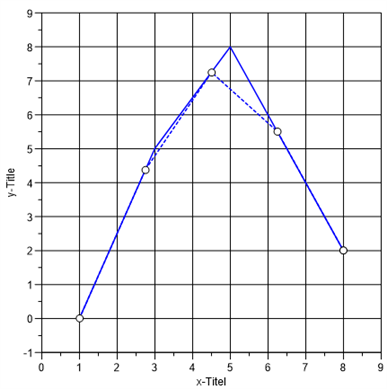# interpol¶

The interpol function executes a linear interpolation or cosine interpolation for the given x-coordinates.

`rvYi = interpol(rvX, rvY, rvXi)`
`rvYi = interpol(rvX, rvY, rvXi, rsMissing)`
`rvYi = interpol(rvX, rvY, rvXi, rsMissing, ssType)`

Return Value

rvYi

Parameters

`rvX`

rvX is a real vector with at least 2 elements. The coordinates must be sorted monoton increasing or decreasing.

`rvY`

rvY is a real vector with at least 2 elements. The number of points must be identical with rvX.

`rvXi`

rvXi is a real vector with at least one point.

`rsMissing`

1.) Values in rvYi >= rsMissing will be ignored in the interpolation. All elements in rvYi will be set to missing for rvYi >= rsMissing. 2.) If an extrapolation is necessary, the extrapolated values in rvYi are set to rsMissing. Default value is 0.

`ssType`

ssType is a string with the text `linear` or `cosine`. Default value is `linear`.

Example```x = [1,3,5,8]
y = [0,5,8,2]
h = plot(x,y)
yi = interpol(x, y, xi = linspace(1, 8, 5));
h = plot(xi, yi, h)
XYSetProps(h, "marker='1' marker-style='circle' curve-style='dash'");

x = [1,3,5,8]
y = [0,5,8,2]
h = plot(x,y)
yi = interpol(x, y, xi = linspace(1, 8, 5));
h = plot(xi, yi, h, 1e10, "cosine")
XYSetProps(h, "marker='1' marker-style='circle' curve-style='dash'");
```

If an error occures interpol returns an error object (see error_create).

```x = [1,3,4, 5,8]
y = [0,5,8,2]
yi = interpol(x, y, [1,2,2]);
if (type(yi) == "error") {
print yi.message;
}
```

History

Version Description
R2018.12 New parameter ssType
4.2.4 rvXi can be non-monotonic.
4.2.0 New